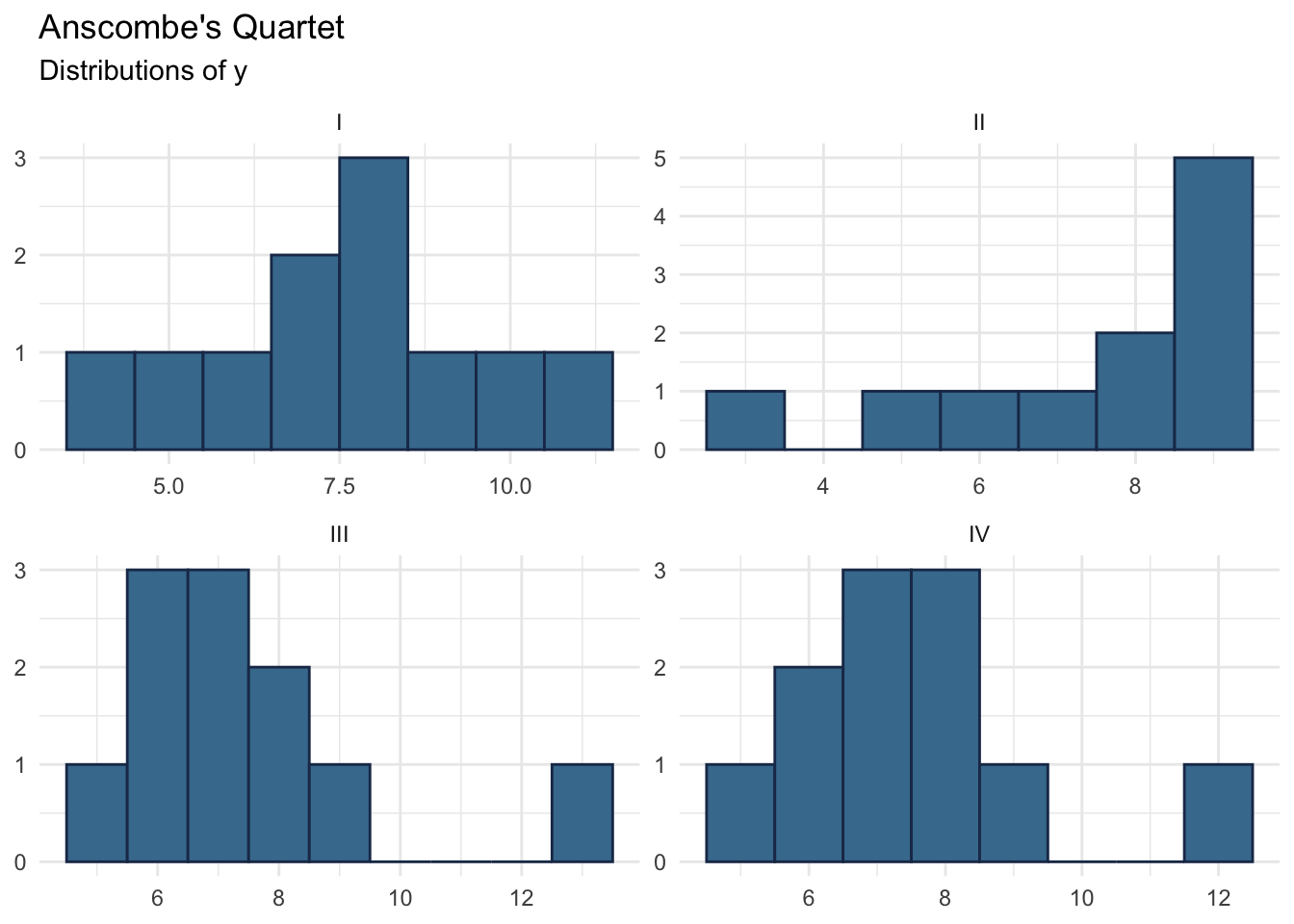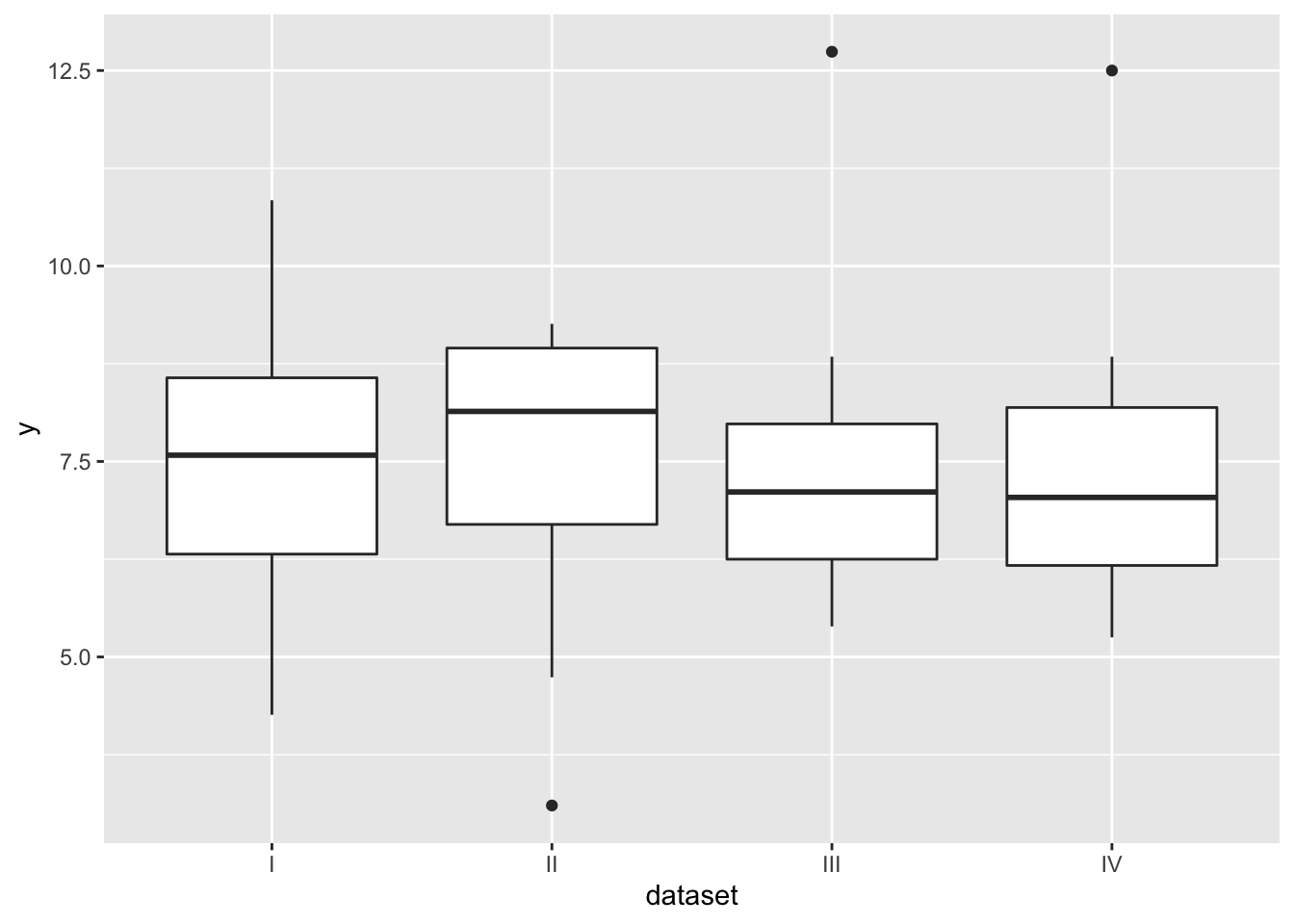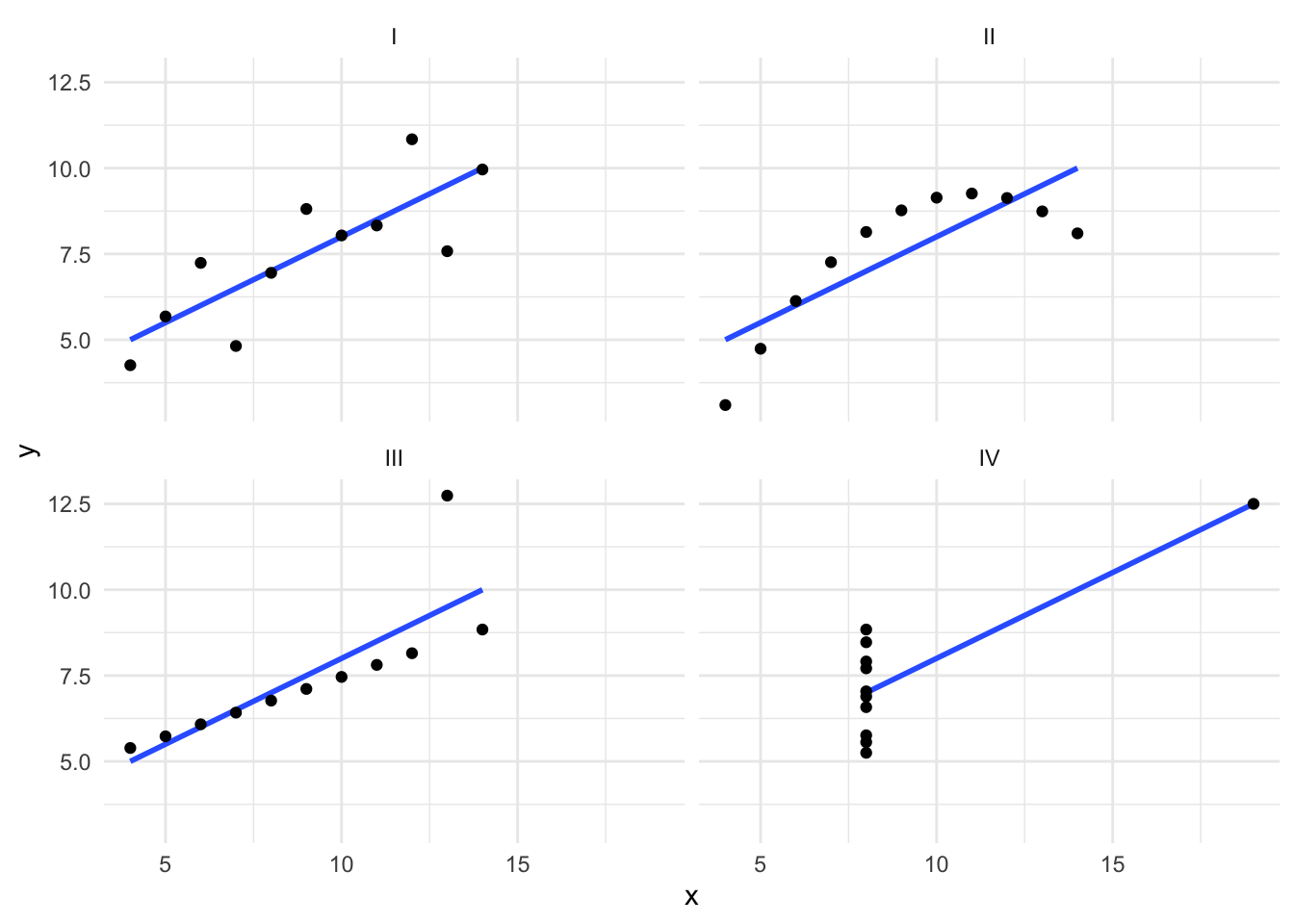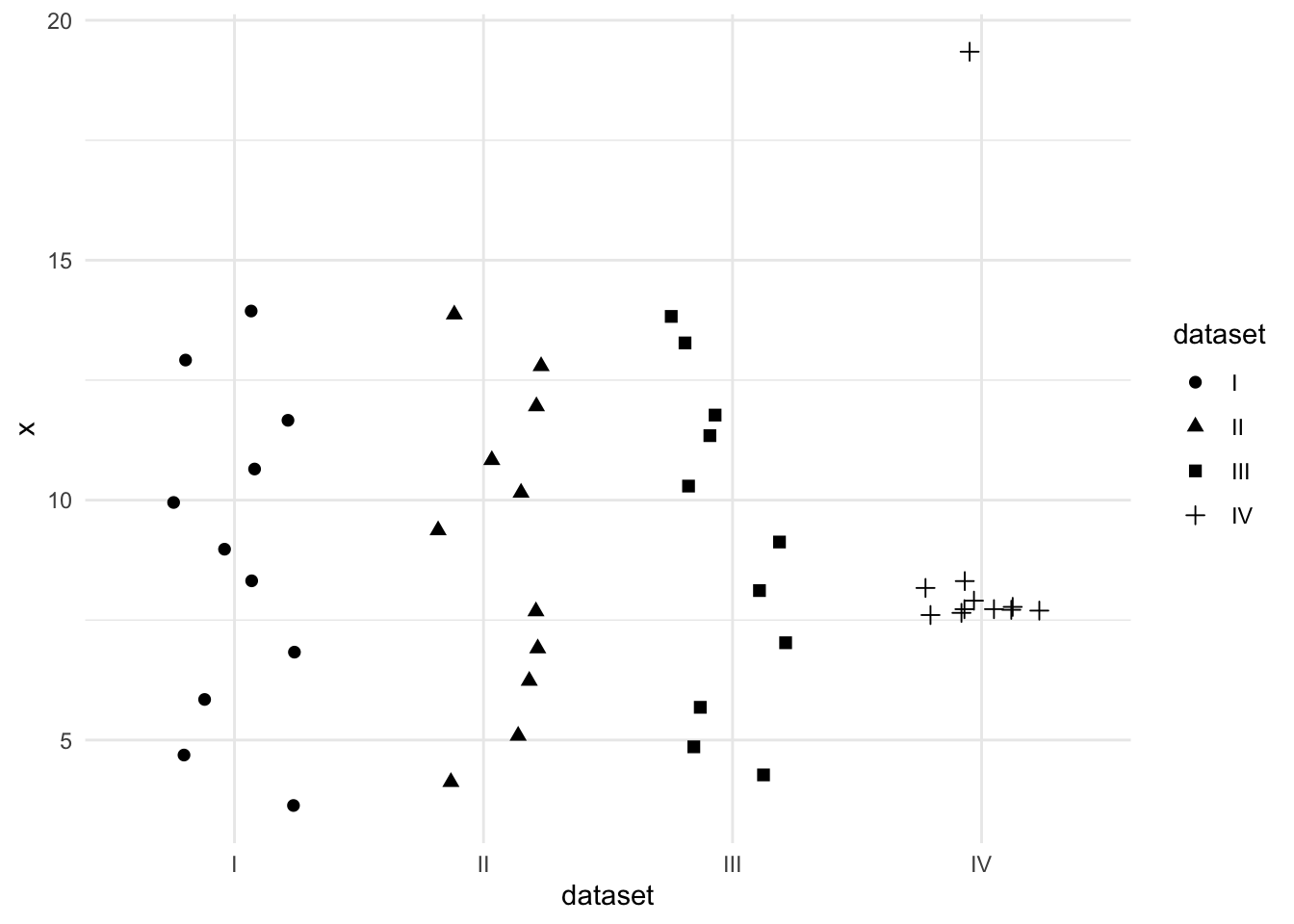## 3.3 Lab Activity

Use the anscombe_long.csv data for this lab activity.

### 3.3.1 Setup

1. If you haven’t already, install ggplot2.
2. Load ggplot2.
3. Import anscombe_long.csv into R as a data.frame named anscombe_long.
4. Convert anscombe_long\$dataset to a factor with levels: 1 = “I”, 2 = “II”, 3 = “III”, and 4 = “IV”.

### 3.3.2 Plots

Write a script to produce each of the following plots. All of them use the anscombe_long.csv data.

### 3.3.3 Plot 1: Histograms### 3.3.4 Plot 2: Boxplot### 3.3.5 Plot 3: Scatter Plot### 3.3.6 Plot 4: Jitter Plot#### Tips for Recreating Plot 4

Identify the aesthetic mappings before you start.

• Which variable is mapped to x?
• Which is mapped to y?
• Are there any other aesthetic mappings?

Use geom_jitter() to plot the points. geom_jitter() is a variant of geom_point() that adds a small amount of random variation to the placement of the points. It’s useful when points would otherwise be overlapping. Note that the jitter is random, so your plot will not match this one exactly. The jitter can also affect the range of the scale axes.

Set the width of geom_jitter() to be equal to 0.25.

This plot uses theme_minimal(). You are free to use whatever theme you prefer.